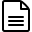# Premium Reports - Inventory Turnover

## Inventory Turnover

These reports are used for measuring how much inventory has been sold and replaced over a given period of time. You can customize this time period via the date range (top right).As Premium Reports, these are available on all Paid Plans of Report Toaster. If you aren't signed up, follow these steps to upgrade your account.

Note that only items that have been sold are included on a Turnover report.

Generally speaking, a good high inventory turnover percentage indicates that a product is selling well and frequently needs to be restocked.
A low inventory turnover percentage indicates that a product is selling infrequently, is overstocked, or both. See this Shopify guide here for more information.

These reports follow a similar format, split up into:
1. Inventory Turnover - Available
This report is ideal for working out turnover based on the quantity sold compared to how much was in stock1. Inventory Turnover - Cost
This report is used to work out turnover based on the cost of goods sold (COGS) compared to the average cost in stock.Please note - Inventory History is only tracked from the time this feature is enabled for your account.
For example, if we enabled the feature for you on 6/20/23, you would have Inventory history from this date onwards.

Here are a few terms to be familiar with in order to understand Inventory Turnover, numbered in the screenshots above:

1.) Available (Start): The number of units/total cost of the product at the start of the time period.
This is determined by the first date on the date range (top right).For example, imagine the report was set to Last 7 Days, today is 6/20/23.
If I had 20 T-shirts in stock on 6/14/23, then  Available (Start) would be 20.

2.) Available (End): The number of units/total cost of the product at the end of the time period.
This is determined by the first date on the date range (top right).If I only had 5 T-shirts left in stock on 6/20/23 at the end of the time period, then Available (End) would be 5.

3.) Total Available (Avg of Range).  This is an average calculated by taking Available (Start) plus Available (End) divided by 2.For example, if the Available (Start) was 20 and the Available (End) was 5, then the Available (Avg of Range) would be (20+5)/2 = 12.5

4.) Inventory Turnover (Available). The Quantity of goods sold over the period divided by the Available (Avg of Range) and then multiplied by 100.For example, if the Quantity sold was 30 and the Available (Avg of Range) was 12.5, then Inventory Turnover (Available) would be 30/12.5 x 100 = 240%

5.) Stock to Sales Ratio. Available (End) divided by Quantity soldFor example, if the Available (End) was 5 and I sold 15, then Stock to Sales Ratio would be 5/20 = 0.25

6.) Total cost (Start): The cost-based inventory value at the start of the time period. This is taken to be Available multiplied by the product's Cost.
This is determined by the first date on the date range (top right).For example, if those 20 T-shirts in stock on 6/14/23 sold for \$20 each, then Total Cost (Start) would be 20 x \$20 = \$400.

7.) Total cost (End): The cost-based inventory value at the end of the time period. This is taken to be Available multiplied by the product's Cost.
This is determined by the first date on the date range (top right).For example, if there were only 5 remaining T-shirts left in stock on 6/20/23 then Total Cost (Start) would be 5 x \$20 = \$100.

8.) Total cost (Avg of Range).  This is an average calculated by taking Total cost (Start) plus Total cost (End) divided by 2.For example, if the Total cost (Start) was \$200 and Total cost (End) was \$100, then the Total cost (Avg of Range) would be (200+100)/2 = \$150

9.) Inventory Turnover (Cost). The total Cost of goods sold (COGS) over the period divided by the Cost (Avg of Range) and then multiplied by 100.For example, if the Cost of goods sold was \$1,500 and the Cost (Avg of Range) was \$500.00, then Inventory Turnover (Available) would be 1500/500 x 100 = 300%

Like all Report Toaster reports, these reports are fully customizable - so you can adjust the date range, add/remove columns, introduce a filter or change the sort to suit your individual needs. See our basic guides here for more info.If you want to see your Inventory History, check out our similar reports here.

• # Related Articles

• ## Premium Report - Stock Report

Stock Reports Stock Reports are used to compare how much of something has been sold vs how much you have in stock at each of your different locations. These reports follow a similar format to the Sell Through Reports, but the available quantity ...
• ## Premium Reports - Inventory History

Inventory History Inventory history reports are used for tracking changes to inventory at either a given point in time (e.g. Today) or over a select period (e.g. Last 30 Days). You can customize this time period via the date range (top right). ...